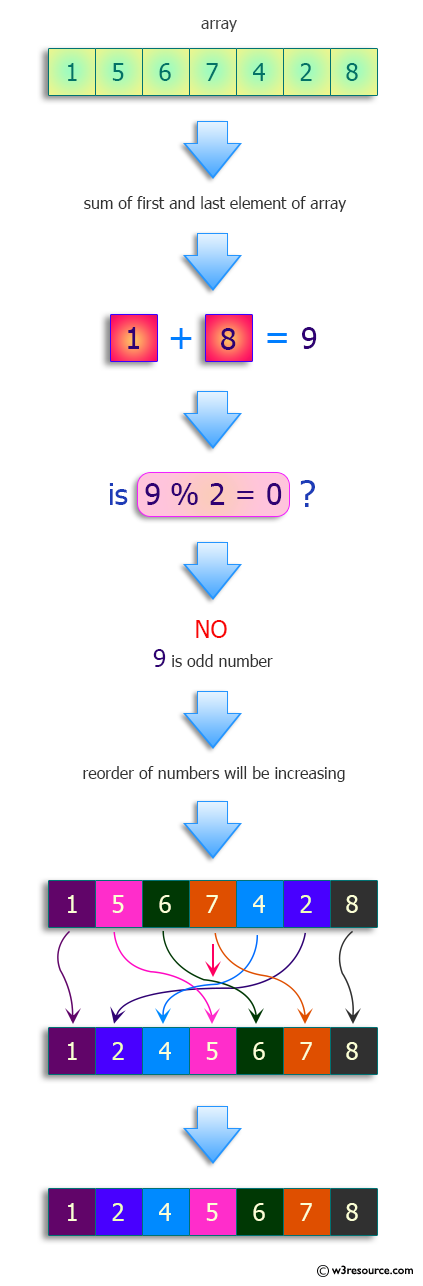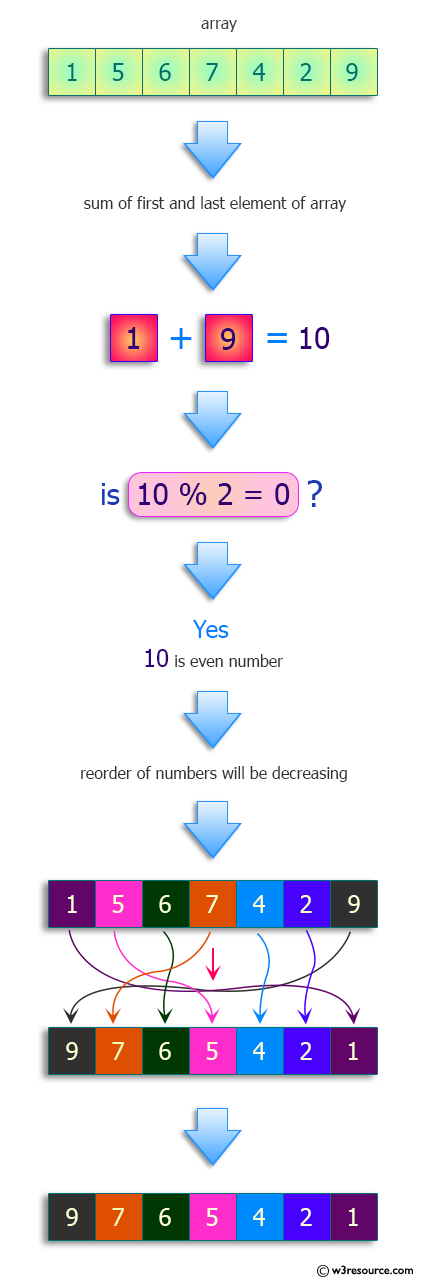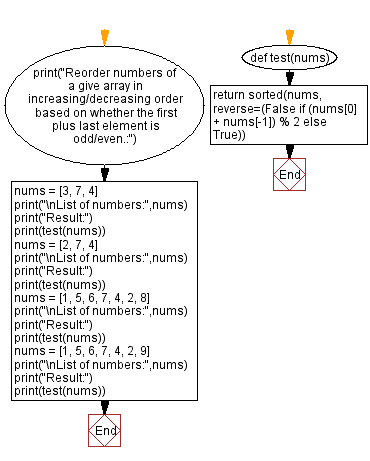﻿ Python: Reorder numbers in increasing/decreasing order based on whether the first plus last element is even/odd - w3resource# Python: Reorder numbers in increasing/decreasing order based on whether the first plus last element is even/odd

## Python Programming Puzzles: Exercise-75 with Solution

Write a Python program to reorder numbers from a give array in increasing/decreasing order based on whether the first plus last element is odd/even.

```Input:
[3, 7, 4]
Output:
[3, 4, 7]

Input:
[2, 7, 4]
Output:
[7, 4, 2]

Input:
[1, 5, 6, 7, 4, 2, 8]
Output:
[1, 2, 4, 5, 6, 7, 8]

Input:
[1, 5, 6, 7, 4, 2, 9]
Output:
[9, 7, 6, 5, 4, 2, 1]
```

Pictorial Presentation:Sample Solution:

Python Code:

``````#License: https://bit.ly/3oLErEI

def test(nums):
return sorted(nums, reverse=(False if (nums + nums[-1]) % 2 else True))
print("Reorder numbers of a give array in increasing/decreasing order based on whether the first plus last element is odd/even.:")
nums = [3, 7, 4]
print("\nList of numbers:",nums)
print("Result:")
print(test(nums))
nums = [2, 7, 4]
print("\nList of numbers:",nums)
print("Result:")
print(test(nums))
nums = [1, 5, 6, 7, 4, 2, 8]
print("\nList of numbers:",nums)
print("Result:")
print(test(nums))
nums = [1, 5, 6, 7, 4, 2, 9]
print("\nList of numbers:",nums)
print("Result:")
print(test(nums))
``````

Sample Output:

```Reorder numbers of a give array in increasing/decreasing order based on whether the first plus last element is odd/even.:

List of numbers: [3, 7, 4]
Result:
[3, 4, 7]

List of numbers: [2, 7, 4]
Result:
[7, 4, 2]

List of numbers: [1, 5, 6, 7, 4, 2, 8]
Result:
[1, 2, 4, 5, 6, 7, 8]

List of numbers: [1, 5, 6, 7, 4, 2, 9]
Result:
[9, 7, 6, 5, 4, 2, 1]
```

Flowchart:## Visualize Python code execution:

The following tool visualize what the computer is doing step-by-step as it executes the said program:

Python Code Editor :

Have another way to solve this solution? Contribute your code (and comments) through Disqus.

What is the difficulty level of this exercise?

Test your Programming skills with w3resource's quiz.

﻿

## Python: Tips of the Day

Clamps num within the inclusive range specified by the boundary values x and y:

Example:

```def tips_clamp_num(num,x,y):
return max(min(num, max(x, y)), min(x, y))
print(tips_clamp_num(2, 4, 6))
print(tips_clamp_num(1, -1, -6))
```

Output:

```4
-1
```